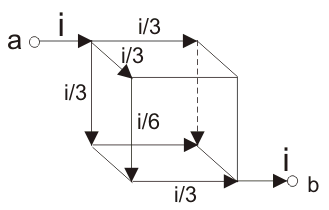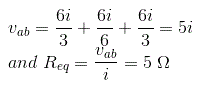# MCQs on Electric Circuits

##### Page 46 of 63. Go to page 1 2 3 4 5 6 7 8 9 10 11 12 13 14 15 16 17 18 19 20 21 22 23 24 25 26 27 28 29 30 31 32 33 34 35 36 37 38 39 40 41 42 43 44 45 46 47 48 49 50 51 52 53 54 55 56 57 58 59 60 61 62 63
01․ If a resistor and an inductor are connected in series across a voltage source. Which two parameters in that circuit increase if frequency of voltage source increases?
VL and Z.
Z and I.
VL and I.
VL and VR.

If frequency of the voltage source increases, the reactance of the inductor increases as because XL = 2πfL and if XL and that causes increase in the total impedance Z, of the circuit, which results decreasing the current I through the circuit. If current is reduced, the voltage drop across the resistor VR is decreased. Again voltage drop across the inductor is the difference of supply voltage and voltage drop across the resistor, hence voltage across inductor VL will increase.

02․ If a resistor and an inductor are connected in series across a voltage source. Which two parameters in that circuit decrease if frequency of voltage source increases?
VL and Z.
VR and I.
Z and I.
VL and VR.

If frequency of the voltage source increases, the reactance of the inductor increases as because XL = 2πfL and if XL and that causes increase in the total impedance Z, of the circuit, which results decreasing the current I through the circuit. If current is reduced, the voltage drop across the resistor VR is decreased. Again voltage drop across the inductor is the difference of supply voltage and voltage drop across the resistor, hence voltage across inductor VL will increase.

03․ If a resistor and a capacitor are connected to form series R - C circuit across a voltage source. If frequency of voltage source increases
the current increases.
the current decreases.
the current remain unaltered.
the current decreases abruptly.

In series RC circuit if supply frequency is increased, the current increases. Because the impedance is capacitive impedance is inversely proportional to frequency. As capacitive impedance decreases with increase in frequency, the overall impedance of the series RC circuit is decreased which results increase in current through the circuit.

04․ A branch of a network is said to be active when it consists of one
resistor.
voltage source.
inductor.
capacitor.

When a branch of a network contains one or more energy sources it is called an active branch.

05․ A branch of a network is said to be passive when it contains
voltmeter.
voltage source.
current source.
battery.

A branch of a network that does not contain one or more energy sources is called a passive branch. Voltmeter is not an energy source but a measuring instrument which measures voltage.

06․ Which of the following is not a bilateral element?
Resistor.
Diode.
Capacitor.
Inductor.

A bilateral element conduct equally well in either directions. Such as resistor and inductor. When the current voltage relation are different for the two directions of current flow, the element is said to be unilateral. Diode is a unilateral element.

07․ Which of the following characteristics is/are attributed to an ideal independent voltage source?
both ‘b’ and ‘d’.
Independent of magnitude of current supplied..
Independent of the magnitude of voltage supplied.
Independent of the direction of flow of current.

Independent ideal voltage source is such a source, which gives fixed voltage, irrespective of magnitude and direction of current flowing through it.

08․ Dependent source of current and voltage are those which have
unidirectional characteristic.
output dependent on input.
independent of any other network variable.
all of the above.

The source whose output voltage or current is a function of the voltage or current in another part of the circuit is called dependent source.

09․ The internal resistance of a practical voltage source is considered to be connected in
series.
parallel.
either parallel or series.
none of the above.

Whenever load is connected to the voltage source, its terminal voltage decreases because of its internal resistance. Hence the internal resistance of a practical voltage source is assumed to be connected in series with the source.

10․ Twelve 6 Ω resistor used as edge to form a cube. The resistance between two diagonally opposite corner of the cube is
6 / 5 Ω.
5 Ω.
6 Ω.
5 / 6 Ω.

The current i will be distributed in the cube branches symmetrically.<<<4445464748>>>